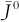### Solution to Application Example 7.11

Calculation of the inverse of $J¯0$, that is. $J¯0−1$.

${\left({\overline{J}}^{0}\right)}^{-1}=\frac{\mathrm{A}\mathrm{d}\mathrm{j}\left({\overline{J}}^{0}\right)}{det\left({\overline{J}}^{0}\right)}\text{,}$where Adj $J¯0$is the adjoint of matrix $J¯0$and det$J¯0$ = |$J¯0$| is the determinant of $J¯0$.

The adjoint of $J¯0$ is obtained by first taking the ...

Get Power Quality in Power Systems and Electrical Machines, 2nd Edition now with the O’Reilly learning platform.

O’Reilly members experience live online training, plus books, videos, and digital content from nearly 200 publishers.